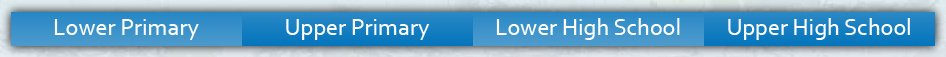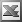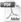Upper Primary Enter keywords to search by topic or worksheet description

Worksheets in this category help students to develop an understanding of perimeter, and also to calculate the perimeter of simple plane shapes.

Worksheet Name Files
Perimeter of a SquarePerimeter and Side Length of a SquarePerimeter of a RectanglePerimeter of Squares & RectanglesPerimeter of a TriangleCircle DimensionsCircumference of a CircleSector PerimeterPerimeter and Circumference ReviewPerimeter of Compound Shapes